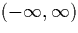Next: Related Work Up: Introduction Previous: Introduction

# Work Performed

The work performed is composed of three distinct parts; finding suitable representations for reals and developing algorithms for the required operations on them, designing and implementing algorithms and a user interface, and analysing the performance of the algorithms and implementation.

We have used two basic representations of reals on the closed interval [-1,1]; streams of signed binary digits, and streams dyadic rationals which are contained in the interval [-1,1] and treated as digits. Many algorithms are easier to define using the dyadic rational representation, but they often perform poorly if the size of the rationals involved grows. The signed binary digit representation does not suffer from this problem, and is easier to use to implement other algorithms because we can use pattern matching with the small, finite set of digits. In fact we use the signed binary representation as the main representation, but observe that for some algorithms, such as division and the functional operations, it is much easier if we take a signed binary input, generate a dyadic output, and then convert the result back into the signed binary representation.

The whole real lineis represented using a mantissa which is a stream of either dyadic or signed binary digits, and an exponent of arbitrary size. A representation of reals as a sequence of nested closed intervals whose end-points are expressed using the signed binary (mantissa, exponent) representation mentioned above is also used because it is useful when implementing algorithms for transcendental functions.

The operations fall into three broad categories; the basic arithmetic operations and ones which convert between representations, high level transcendental functions, and the functional operations. Many of the algorithms for the basic operations are already known, but in this work we develop algorithms computing the division of two numbers, for the multiplication of two signed binary streams, and for computing the limits of Cauchy sequences and hence many transcendental functions.

The algorithm for the multiplication of signed binary developed streams does so working solely with signed binary digits. The novel (but considerably simpler) approach originally proposed during personal communications with Alex Simpson and Martín Escardó was to take a signed binary digit input, generate output using the dyadic digits, and then convert the result back into a signed binary representation.

The division algorithm developed and proved is more complex than the algorithms for the other basic arithmetic operations. It is also considerably more efficient than an alternative approach tested which found the reciprocal of the denominator by computing the limit of an iterative approximation of reciprocal, and then multiplying this by the numerator.

The new algorithm designed for the computation of limits of Cauchy sequences is a development of an idea originally proposed by Martín Escardó (personal communication). It uses the representation of reals as a sequence of nested closed intervals whose end-points use the signed binary (mantissa, exponent) representation. Given a suitable Cauchy sequence for which we know the rate (or sometimes just the manner) of convergence to its limit, we can generate a sequence of nested intervals of this form. The algorithm allows us to then convert this sequence of nested intervals representation into a single numeral which representing the limit of the original Cauchy sequence. We give a description of this algorithm, and an proof of correctness.

The algorithm for the computation of the limits of Cauchy sequence forms the basis of the algorithms for transcendental functions. Many transcendental functions can be expressed very easily as the limits of Cauchy sequences, and once we have found such a sequence we can use the approach described above directly to implement an algorithm to compute the function. The set of transcendental functions which we implement in the calculator includes the basic trigonometric and logarithmic functions, but this set of available functions could be extended with very little additional effort to include more transcendental functions using this approach.

Also implemented here are Alex Simpson's algorithms for the functional operations integration and function minimum and maximum . In particular, this includes the first implementation of these algorithms on the whole real line.

We implement the calculator using the functional programming language Haskell. The implementation can be loosely divided into the modules containing the core algorithms, and a simple text based user interface which is used to demonstrate them.

Finally we analyse the algorithms and implementation. This allows us to evaluate the approach and discuss the performance of the algorithms. Experimentation is used to test hypotheses made in the theoretical analysis, and allows us to assess the performance of the implementation as a whole.

We examine the basic algorithms to determine the lookahead (the number of digits of input are required to obtain n digits of output), whether the algorithm branches (spawns additional stream operations as it generates output digits), and whether the size of the representation of dyadic rational style digits swells in algorithms which output dyadic digit streams. These results can then be used to examine expressions involving sequences of operations. We make observations about the lookahead required for the iterated logistic map and the effect of changing the structure of expressions can have on the lookahead.

The experimentation confirms some of the theoretical predications and demonstrates the real behaviour of the algorithms for which we can determine a minimum and maximum lookahead, but for which these are different and the maximum lookahead is not always required. It also illustrates the problem of the dyadic digit representation size swelling and its effect on performance. Lastly we show that although the performance of this implementation is adequate for simple computations, this particular implementation is probably too slow to be applied to applications involving more complex computations. We discuss why this might be so.Next: Related Work Up: Introduction Previous: Introduction
Martin Escardo
5/11/2000